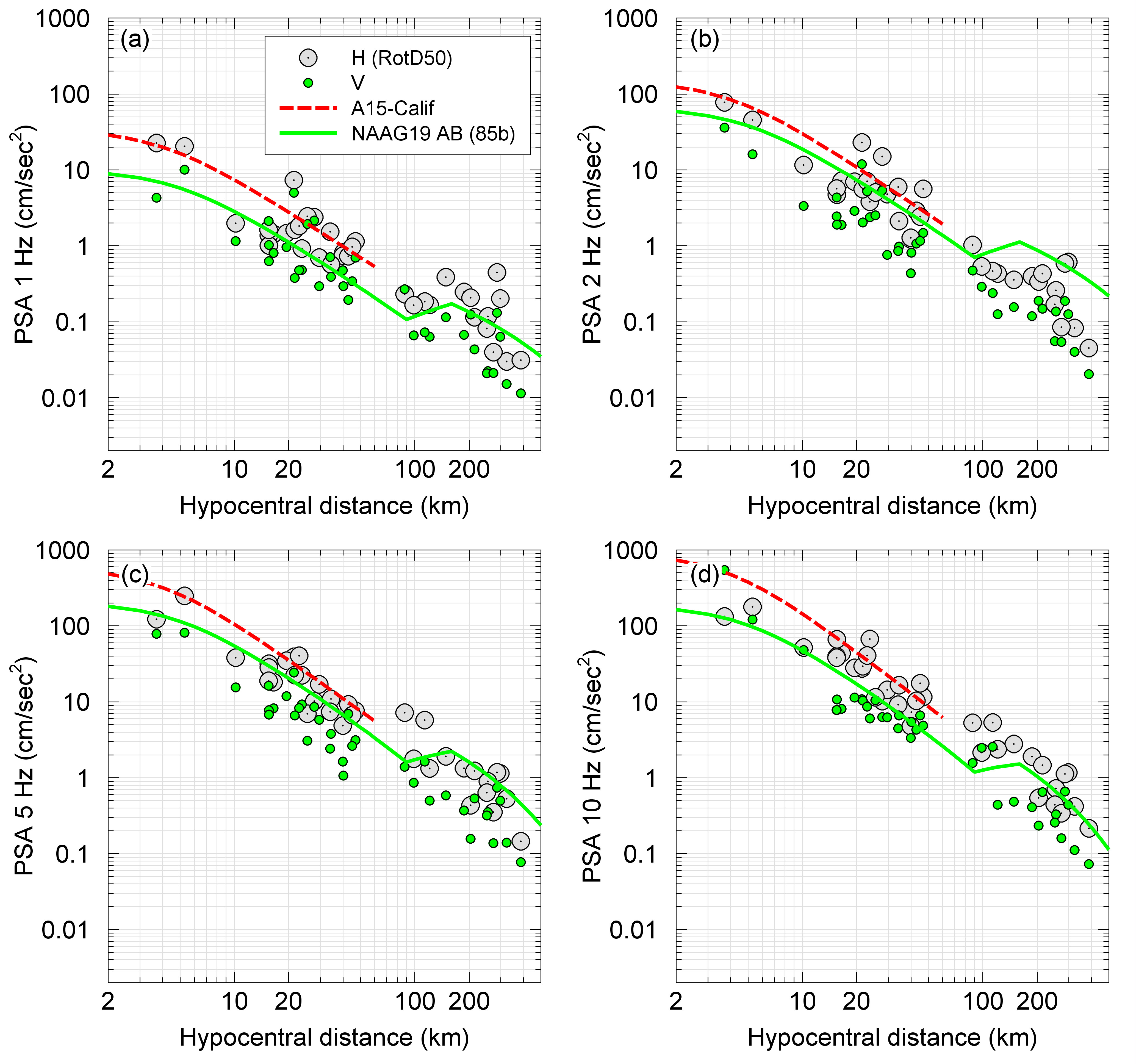# Ground Motion Analysis

• Data processing and calculation of ground motion amplitudes at each station
• Linear and non-linear amplification study
• Effect of rupture direction on ground motion amplitudes
• Evaluation and estimation of appropriate ground motion prediction equation and calibration to data
• Scenario and real-data ShakeMaps
• Waveform selection and simulation for engineering applications